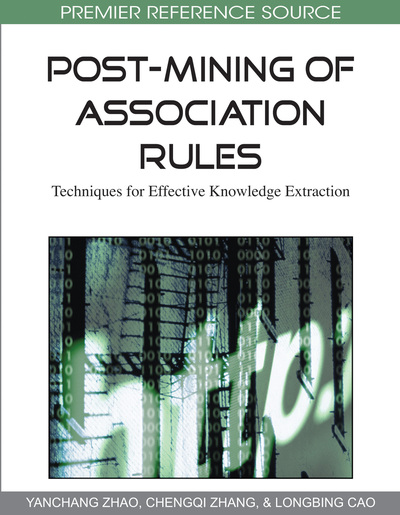# Semantics-Based Classification of Rule Interestingness Measures

Julien Blanchard (Polytechnic School of Nantes University, France), Fabrice Guillet (Polytechnic School of Nantes University, France) and Pascale Kuntz (Polytechnic School of Nantes University, France)
DOI: 10.4018/978-1-60566-404-0.ch004
Available
\$33.75
List Price: \$37.50
10% Discount:-\$3.75
TOTAL SAVINGS: \$3.75

## Abstract

Assessing rules with interestingness measures is the cornerstone of successful applications of association rule discovery. However, as numerous measures may be found in the literature, choosing the measures to be applied for a given application is a difficult task. In this chapter, the authors present a novel and useful classification of interestingness measures according to three criteria: the subject, the scope, and the nature of the measure. These criteria seem essential to grasp the meaning of the measures, and therefore to help the user to choose the ones (s)he wants to apply. Moreover, the classification allows one to compare the rules to closely related concepts such as similarities, implications, and equivalences. Finally, the classification shows that some interesting combinations of the criteria are not satisfied by any index.
Chapter Preview
Top

## Introduction

Most of association rule mining algorithms are unsupervised algorithms, i.e. they do not need any endogenous variable but search all the valid associations existing in the data. This makes the main interest of association rules, since the algorithms can discover relevant rules that the user didn’t even think of beforehand. However, the unsupervised nature of association rules causes their principal drawback too: the number of rules generated increases exponentially with the number of variables. Then a very high number of rules can be extracted even from small datasets.

To help the user to find relevant knowledge in this mass of information, many Rule Interestingness Measures (RIM) have been proposed in the literature. RIMs allow one to assess, sort, and filter the rules according to various points of view. They are often classified into two categories: the subjective (user-oriented) ones and the objective (data-oriented) ones. Subjective RIMs take into account the user’s goals and user’s beliefs of the data domain (Silberschatz & Tuzhilin, 1996; Padmanabhan & Tuzhilin, 1999; Liu et al., 2000). On the other hand, the objective RIMs do not depend on the user but only on objective criteria such as data cardinalities or rule complexity. In this chapter, we are interested in the objective RIMs. This category is very heterogeneous: one can find both elementary measures based on frequency and sophisticated measures based on probabilistic models, as well as information-theoretic measures or statistical similarity measures. In practice, the use of RIMs is problematic since:

• The RIMs are too numerous, and sometimes redundant (Bayardo & Agrawal, 1999; Tan et al., 2004; Blanchard et al., 2005a; Huynh et al., 2006; Lenca et al., 2007).

• The meanings of the RIMs are often unclear, so that it is hard to know precicely what is measured.

• Finally, choosing the RIMs to apply for a given study remains a difficult task for the user.

The main contribution of this chapter is to present a novel and useful classification of RIMs according to three criteria: the subject, the scope, and the nature of the measure. These criteria seem to us essential to grasp the meaning of the RIMs, and therefore to help the user to choose the ones (s)he wants to apply. Moreover, the classification allows one to compare the rules to closely related concepts such as similarities, implications, and equivalences. Finally, the classification shows that some interesting combinations of the criteria are not satisfied by any index.

The remainder of the chapter is organized as follows. In the next section, after introducing the notations, we formalize the concepts of rule and interestingness measure, and then take inventory of numerous measures traditionally used to assess rules. Section 3 defines the three classification criteria, presents our classification of rule interestingness measures, and describes two original measures that we specifically developed to complement the classification. Section 4 discusses the related works. Finally, we give our conclusion in section 5.

Top

## Rules And Interestingness Measures

### Notations

We consider a set O of n objects described by boolean variables. In the association rule terminology, the objects are transactions stored in a database, the variables are called items, and the conjunctions of variables are called itemsets.

Let a be a boolean variable which is either an itemset, or the negation of an itemset1. The variable a* is the negation of a. We note A the set of objects that verify a, and na the cardinality of A. The complementary set of A in O is the set A* with cardinality na*. The probability of the event “a is true” is noted P(a). It is estimated by the empirical frequency: P(a)=na/n.

In the following, we study two boolean variables a and b. The repartition of the n objects in O with regard to a and b is given by the contingency Figure 1, where the value nab is the number of objects that verify both a and b.

## Complete Chapter List

Search this Book:
Reset